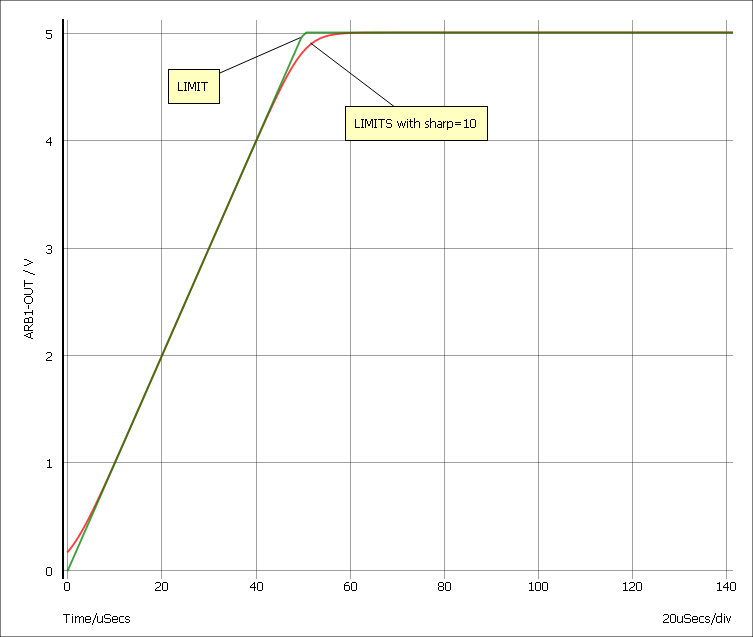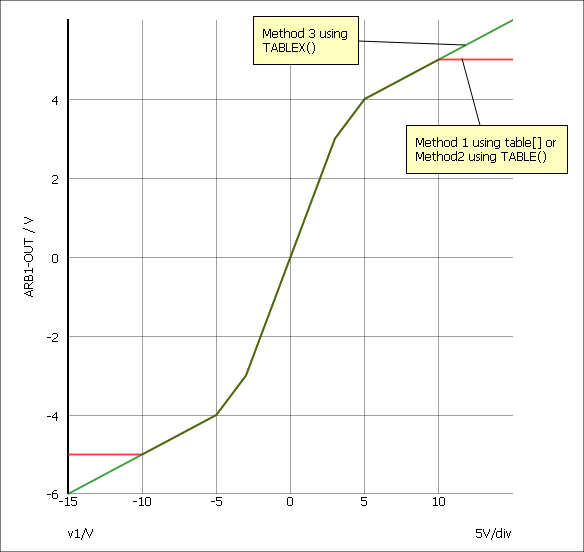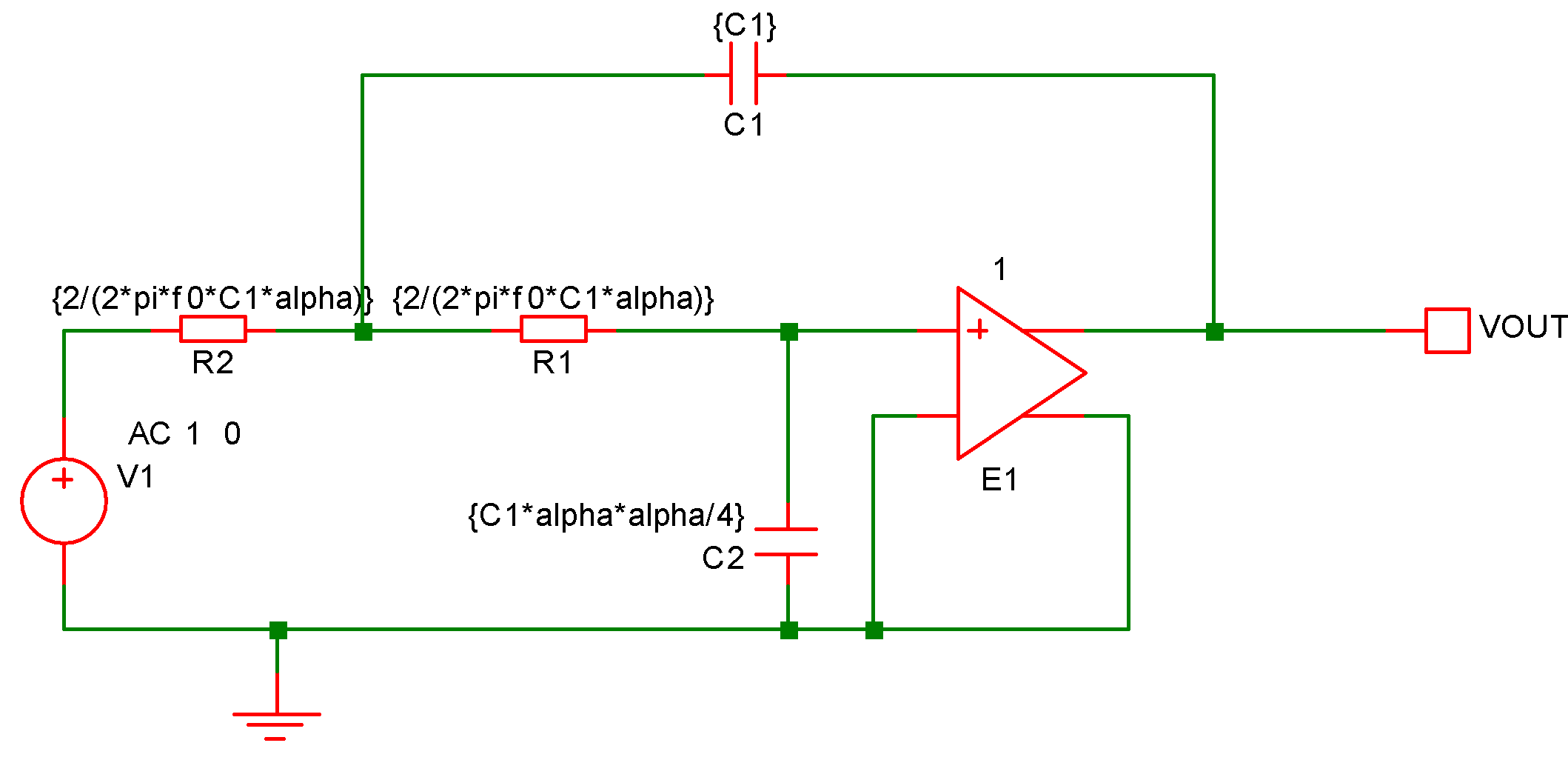Using Expressions

In this topic:

Overview

Expressions consist of arithmetic operators, functions, variables and constants and may be employed in the following locations:

• As device parameters
• As model parameters
• To define a variable (see .PARAM) which can itself be used in an expression.
• As the governing expression used for arbitrary sources.

They have a wide range of uses. For example:

• To define a number of device or model parameters that depend on some common characteristic. This could be a circuit specification such as the cut-off frequency of a filter or maybe a physical characteristic to define a device model.
• To define tolerances used in Monte Carlo analyses.
• Used with an arbitrary source, to define a non-linear device.

Using Expressions for Device Parameters

Device or instance parameters are placed on the device line. For example the length parameter of a MOSFET, L, is a device parameter. A MOSFET line with constant parameters might be:

M1 1 2 3 4 MOS1 L=1u W=2u

L and W could be replaced by expressions. For example:

M1 1 2 3 4 MOS1 L={LL-2*EDGE} W={WW-2*EDGE}

Device parameter expressions must usually be enclosed with either single quotation marks ( ' ) double quotation marks ( " ) or braces ( '{' and '}' ). The expression need not be so enclosed if it consists of a single variable. For example:

.PARAM LL=2u WW=1u
M1 1 2 3 4 MOS1 L=LL W=WW

Using Expressions for Model Parameters

The rules for using expressions for device parameters also apply to model parameters. E.g.

.MODEL N1 NPN IS=is BF={beta*1.3}

Expression Syntax

Expressions generally comprise the following elements:

• Circuit variables
• Parameters
• Constants.
• Operators
• Functions
• Look up tables
These are described in the following sections.

Circuit Variables

Circuit variables may only be used in expressions used to define arbitrary sources and to define variables that themselves are accessed only in arbitrary source expressions.

Circuit variables allow an expression to reference voltages and currents in any part of the circuit being simulated.

Voltages are of the form:

V(node_name1)
OR
V(node_name1, node_name2)

Where node_name1 and node_name2 are the name of the node carrying the voltage of interest. The second form above returns the difference between the voltages on node_name1 and node_name2. If using the schematic editor nodenames are normally allocated by the netlist generator. For information on how to display and edit the schematic's node names, refer to Displaying Net and Pin Names.

Currents are of the form:

I(source_name)

Where source_name is the name of a voltage source carrying the current of interest. The source may be a fixed voltage source, a current controlled voltage source, a voltage controlled voltage source or an arbitrary voltage source. It is legal for an expression used in an arbitrary source to reference itself e.g.:

B1 n1 n2 V=100*I(B1)

Implements a 100 ohm resistor.

Parameters

These are defined using the .PARAM statement. For example:

.PARAM res=100
B1 n1 n2 V=res*I(B1)

Also implements a 100 ohm resistor.

Circuit variables may be used .PARAM statements, for example:

.PARAM VMult = { V(a) * V(b) }
B1 1 2 V = Vmult + V(c)

Parameters that use circuit variables may only be used in places where circuit variables themselves are allowed. So, they can be used in arbitrary sources and they may be used to define the resistance of an Hspice style resistor which allows voltage and current dependence. (See Resistor - Hspice Compatible). They may also of course be used to define further parameters as long as they too comply with the above condition.

Built-in Parameters

A number of parameter names are assigned by the simulator. These are:

 Parameter name Description TIME Resolves to time for transient analysis. Resolves to 0 otherwise including during the pseudo transient operation point algorithm. Note that this may only be used in an arbitrary source expression TEMP Resolves to current circuit temperature in Celsius HERTZ Resolves to frequency during AC sweep and zero in other analysis modes PTARAMP Resolves to value of ramp during pseudo transient operating point algorithm if used in arbitrary source expression. Otherwise this value resolves to 1.

Constants

Apart from simple numeric values, arbitrary expressions may also contain the following built-in constants:

 Constant name Value Description PI 3.14159265358979323846 $\pi$ E 2.71828182845904523536 e TRUE 1.0 FALSE 0.0 ECHARGE 1.6021918e-19 Charge on an electron in coulombs BOLTZ 1.3806226e-23 Boltzmann's constant
If the simulator is run from the front end in GUI mode, it is also possible to access variables defined on the Command Shell command line or in a script. The variable must be global and enclosed in braces. E.g.
B1 n1 n2 V = V(n3, n3) * { global:amp_gain }

amp_gain could be defined in a script using the LET command. E.g. "Let global:amp_gain = 100"

Operators

These are listed below and are listed in order of precedence. Precedence controls the order of evaluation. So 3*4 + 5*6 = (3*4) + (5*6) = 42 and 3+4*5+6 = 3 + (4*5) + 6 = 29 as '*' has higher precedence than '+'.

 Operator Description ~ ! - Digital NOT, Logical NOT, Unary minus ^ or ** Raise to power. *, / Multiply, divide +, - Plus, minus >=, <=, > < Comparison operators ==, != or <> Equal, not equal & Digital AND (see below) | Digital OR (see below) && Logical AND ^^ Exclusive OR || Logical OR test ? true_expr : false_expr Ternary conditional expression (see below)

Comparison, Equality and Logical Operators

These are Boolean in nature either accepting or returning Boolean values or both. A Boolean value is either TRUE or FALSE. FALSE is defined as equal to zero and TRUE is defined as not equal to zero. So, the comparison and equality operators return 1.0 if the result of the operation is true otherwise they return 0.0.

The arguments to equality operators should always be expressions that can be guaranteed to have an exact value e.g. a Boolean expression or the return value from functions such as SGN. The == operator, for example, will return TRUE only if both arguments are exactly equal. So the following should never be used:

v(n1)==5.0

v(n1) may not ever be exactly 5.0. It may be 4.9999999999 or 5.00000000001 but only by chance will it be 5.0.

These operators are intended to be used with the IF() function described in Ternary Conditional Expression.

Digital Operators

These are now considered obsolete and should not be used in new model designs. They may be removed from future versions of SIMetrix.

These are the operators '&', '|' and '~'. These were introduced in old SIMetrix version as a simple means of implementing digital gates in the analog domain. Their function has largely been superseded by gates in the event driven simulator.

Ternary Conditional Expression

This is of the form:

test_expression ? true_expression : false_expression

The value returned will be true_expression if test_expression resolves to a non-zero value, otherwise the return value will be false_expression. This is functionally the same as the IF() function described in the functions table below.

Functions

 Function Description ABS(x) $|x|$ ACOS(x), ARCCOS(x) $cos^{-1}(x)$ ACOSH(x) $cosh^{-1}(x)$ ASIN(x), ARCSIN(x) $sin^{-1}(x)$ ASINH(x) $sinh^{-1}(x)$ ATAN(x), ARCTAN(x) $tan^{-1}(x)$ ATAN2(x,y) $tan^{-1}(x/y)$. Valid if y=0 ATANH(x) $tanh^{-1}(x)$ COS(x) $cos(x)$ COSH(x) $cosh(x)$ DDT(x) $dx/dt$ EXP(x) $e^{x}$ FLOOR(x), INT(x) Next lowest integer of $x$. IF(cond, x, y[, maxslew]) if cond is TRUE $result=x$ else $result=y$. If $maxslew>0$ the rate of change of the result will be slew rate controlled. See IF() Function. IFF(cond, x, y[, maxslew]) As IF(cond, x, y, maxslew) LIMIT(x, lo, hi) if $xhi$ $result=hi$ else $result=x$ LIMITS(x, lo, hi, sharp) As LIMIT but with smoothed corners. The 'sharp' parameter defines the abruptness of the transition. A higher number gives a sharper response. LIMITS gives better convergence than LIMIT. See LIMITS() Function. below LN(x) $ln(x)$ If $x<10^{-100}$ $result=-230.2585093$ LNCOSH(x) $ln(cosh(x))$ = $\int tanh(x) dx$ LOG(x) $log_{10}(x)$. If $x<10^{-100}$ $result=-100$ LOG10(x) $log_{10}(x)$. If $x<10^{-100}$ $result=-100$ MAX(x, y) Returns larger of $x$ and $y$ MIN(x,y) Returns smaller of $x$ and $y$ PWR(x,y) $x^y$ PWRS(x,y) $x>=0$: $x^y$, $x<0$: $-x^y$ SDT(x) $\int x dt$ SGN(X) $x>0$: 1, $x<0$: -1, $x=0$: 0 SIN(x) $sin(x)$ SINH(x) $sinh(x)$ SQRT(x) $x>=0$: $\sqrt{x}$, $x<0$: $\sqrt{-x}$ STP(x) $x<=0$: 0, $x>0$: 1 TABLE(x,xy_pairs) lookup table. Refer to Lookup tables TABLEX(x,xy_pairs) Same as TABLE except end points extrapolated. Refer to Lookup tables TAN(x) $tan(x)$ TANH(x) $tanh(x)$ U(x) as STP(x) URAMP(x) $x<0$: 0, $x>=0$: $x$

Monte Carlo Distribution Functions

To specify Monte Carlo tolerance for a model parameter, use an expression containing one of the following functions:

 Name Distribution Lot? DISTRIBUTION2 User defined distribution No DISTRIBUTION2L User defined distribution Yes UD2 Alias for DISTRIBUTION2() No UD2L Alias for DISTRIBUTION2L() Yes GAUSS Gaussian No GAUSSL Gaussian Yes GAUSSTRUNC Truncated Gaussian No GAUSSTRUNCL Truncated Gaussian Yes UNIF Uniform No UNIFL Uniform Yes UNIF2 Uniform No UNIFL2 Uniform Yes WC Worst case No WCL Worst case Yes WC2 Worst case No WCL2 Worst case Yes GAUSSE Gaussian logarithmic No GAUSSEL Gaussian logarithmic Yes UNIFE Uniform logarithmic No UNIFEL Uniform logarithmic Yes WCE Worst case logarithmic No WCEL Worst case logarithmic Yes
A full discussion on the use of Monte Carlo distribution functions is given in Specifying Tolerances.

IF() Function

IF(condition, true-value, false-value[, max-slew])
The result is:

if condition is non-zero, result is true-value, else result is false-value.

If max-slew is present and greater than zero, the result will be slew-rate limited in both positive and negative directions to the value of max-slew.

In some situations, for example if true-value and false-value are constants, the result of this function will be discontinuous when condition changes state. This can lead to non-convergence as there is no lower bound on the time-step. In these cases a max-slew parameter can be included. This will limit the slew rate so providing a controlled transition from the true-value to the false-value and vice-versa.

If the option setting DISCONTINUOUSIFSLEWRATE is non-zero, SIMetrix will automatically apply a max-slew parameter to all occurrences of the IF() function if both true-value and false-value are constants. This provides a convenient way of resolving convergence issues with third-party models that make extensive use of discontinuous if expressions. Note that the DISCONTINUOUSIFSLEWRATE option is also applied to conditional expressions using the C-style condition ? true-value : false-value syntax.

LIMITS() Function

LIMITS(x, low, high, sharp)
The LIMITS() function is similar to LIMIT but provides a smooth response at the corners which leads to better convergence behaviour. The behaviour is shown belowThe LIMITS function follows this equation:

LIMITS(x, low, high, sharp) = 0.5*((ln(cosh(v1))-ln(cosh(v2)))/v3 +(low+high))

Where
• v1 = sharp/(high-low)*(x-low)
• v2 = sharp/(high-low)*(x-high)
• v3 = sharp/(high-low)

Look-up Tables

Expressions may contain any number of look-up tables. This allows a transfer function of a device to be specified according to - say - measured values without having to obtain a mathematical equation. Look-up tables are specified in terms of x, y value pairs which describe a piece-wise linear transfer function. There are three ways to create a lookup table:
Method 1 Syntax in form: TABLE[xy_pairs](input_expression).
 xy_pairs A sequence of comma separated pairs of constant values that define the input and output values of the table. For each pair, the first value is the x or input value and the second is the y or output value. Only explicit numeric constants may be used. Even internal constants such as PI may not be used. input_expression Expression defining the input or x value of the table.
Method 2 Syntax in form: TABLE(input_expression, xy_pairs).
 input_expression Expression defining the input or x value of the table. xy_pairs A sequence of comma separated pairs of values that define the input and output values of the table. For each pair, the first value is the x or input value and the second is the y or output value. Unlike method 1, values do not need to be literal constants and may be expressions containing parameters and circuit variables that reference voltages and currents in the circuit. The TABLE function retains the value of the end point for x values beyond the range of the table. For example, if the last point for the TABLE function is (10,5) then any input greater than 10 will give an output of 5. If any value references a circuit variable, the expression in which the TABLE function is used must ultimately be evaluated in an arbitrary source
Method 3 Syntax in form: TABLEX(input_expression, xy_pairs).
 input_expression Expression defining the input or x value of the table. xy_pairs Same as method 2 except that out-of-range points are extrapolated from the final segment. See Table Lookup Example

Method 1 is more efficient at handling large tables (hundreds of values). However, method 2 is generally more flexible and is the recommended choice for most applications. Method 2 is also compatible with other simulators whereas method 1 is proprietary to SIMetrix.

For an example see Table Lookup Example

Examples

Table Lookup Example

The following arbitrary source definition implements a soft limiting function

Using method 1:
B1 n2 n3 V=table[-10, -5, -5, -4, -4, -3.5, -3, -3, 3, 3, 4,
+ 3.5, 5, 4, 10, 5](v(N1))
Using method 2:
B1 n2 n3 V=TABLE(v(N1), -10, -5, -5, -4, -4, -3.5, -3, -3, 3, 3, 4,
+ 3.5, 5, 4, 10, 5)
Using method 3:
B1 n2 n3 V=TABLEX(v(N1), -10, -5, -5, -4, -4, -3.5, -3, -3, 3, 3, 4,
+ 3.5, 5, 4, 10, 5)

The resulting transfer functions are illustrated in the following picture:Parameter Example

It is possible to assign expressions to component values which are evaluated when the circuit is simulated. This has a number of uses. For example you might have a filter design for which several component values affect the roll off frequency. Rather than recalculate and change each component every time you wish to change the roll of frequency it is possible to enter the formula for the component's value in terms of this frequency.The above circuit is that of a two pole low-pass filter. C1 is fixed and R1=R2. The design equations are:

R1=R2=2/(2*pi*f0*C1*alpha)
C2=C1*alpha*alpha/4

where f0 is the cut off frequency and alpha is the damping factor.

The complete netlist for the above circuit is:

V1 V1_P 0 AC 1 0
C2 0 R1_P {C1*alpha*alpha/4}
C1 VOUT R1_N {C1}
E1 VOUT 0 R1_P 0 1
R1 R1_P R1_N {2/(2*pi*f0*C1*alpha)}
R2 R1_N V1_P {2/(2*pi*f0*C1*alpha)}

Before running the above circuit you must assign values to the variables. This can be done by one of three methods:

• With the .PARAM statement placed in the netlist.
• With Let command from the command line or from a script. (If using a script you must prefix the parameter names with global:)
• By sweeping the value with using parameter mode of a swept analysis (see General Sweep Specification) or multi-step analysis (see Multi Step Analyses).
Expressions for device values must be entered enclosed in curly braces ('{' and '}').

Suppose we wish a 1kHz roll off for the above filter.

Using the .PARAM statement, add these lines to the netlist

.PARAM f0 1k
.PARAM alpha 1
.PARAM C1 10n

Using the Let command, you would type:

Let f0=1k
Let alpha=1
Let C1=10n
If you then wanted to alter the damping factor to 0.8 you only need to type in its new value:
Let alpha=0.8

then re-run the simulator.

To execute the Let commands from within a script, prefix the parameter names with global:. E.g. "Let global:f0=1k"

In many cases the .PARAM approach is more convenient as the values can be stored with the schematic.

Optimisation

Overview

An optimisation algorithm may be enabled for expressions used to define arbitrary sources and any expression containing a swept parameter. This can improve performance if a large number of such expressions are present in a design.

The optimiser dramatically improves the simulation performance of the power device models developed by Infineon. See Optimiser Performance.

Why is it Needed?

The simulator's core algorithms use the Newton-Raphson iteration method to solve non-linear equations. This method requires the differential of each equation to be calculated and for arbitrary sources, this differentiation is performed symbolically. So as well as calculating the user supplied expression, the simulator must also evaluate the expression's differential with respect to each dependent variable. These differential expressions nearly always have some sub-expressions in common with sub-expressions in the main equation and other differentials. Calculation speed can be improved by arranging to evaluate these sub-expressions only once. This is the main task performed by the optimiser. However, it also eliminates factors found on both the numerator and denominator of an expression as well as collecting constants together wherever possible.

Using the Optimiser

The optimiser is automatically enabled and no action is required to make use of it. If desired, it can be disabled using:

.OPTIONS optimise=0

Optimiser Performance

The optimisation algorithm was added to SIMetrix primarily to improve the performance of some publicly available power device models from Infineon. These models make extensive use of arbitrary sources and many expressions are defined using .FUNC.

The performance improvement gained for these model is in some cases dramatic. For example a simple switching PSU circuit using a SGP02N60 IGBT ran around 5 times faster with the optimiser enabled and there are other devices that show an even bigger improvement.

Accuracy

The optimiser simply changes the efficiency of evaluation and doesn't change the calculation being performed in any way. However, performing a calculation in a different order can alter the least significant digits in the final result. In some simulations, these tiny changes can result in much larger changes in circuit solution. So, you may find that switching the optimiser on and off may change the results slightly.